News

30.07.2019  Уважаемые пользователи, прошу для заказа лицензии обращаться напрямую по адресу support@visual-pvt.com, заявка через сайт временно не работает

02.03.2019  VisualPipe. В версии 1.0.67 добавлен выбор расчетных величин (массовый расход, давление на входе, давление на выходе)

18.02.2019  VisualPVT. An English version of the site is available (see the RUS / ENG options of the main menu)

All news

## Documentation (the section is expanding, watch for updates)

Introduction

Quick Start Instructions

Building the tree of correlations

Up

## Introduction

The VisualPVT software is designed to calculate thermophysical properties of oil, water and gas based on correlation dependences (correlations) under various temperature and pressure conditions. VisualPVT includes about 150 correlations applicable to calculation of such properties as bubble point pressure, gas-oil ratio, formation volume factor, compressibility, density, viscosity, interfacial tension, heat capacity, thermal conductivity, etc. The need to use correlations arises in modeling of reservoir fluids and technological processes under the compositional data constrains. Each correlation is valid for a specific geographic region or experimental sample as defined by an author of a correlation. Relevant validity limits are displayed by during the software operation.

The input data for correlation dependent calculations are usually pressure, temperature, oil specific gravity, and bubble point gas-oil ratio. The unique capability of VisualPVT is recalculation of input properties, for which a user has no experimental data, using other correlations, thereby forming the composite correlations that most closely correspond to the PVT properties interdependence.

Each correlation is open for on-the-fly modifications via the embedded VBA-based editor requiring no programming skills. It enables expanding the correlation list by users and free correlations exchange between users.

The key functions of VisualPVT: building the correlations tree, supplemented with calculations at various temperature and pressure conditions, a graphical representation of multiple correlations on the same graph, extensible measurement units converter, autosave function, Russian and English interfaces.

The VisualPVT project is being actively developed. The project is open to suggestions for improvement and to adaptation for other application areas (including a different set of calculated properties and dependences).

Up

## Quick Start Instructions

The main window consists of five functional Boxes that define five basic steps of performing the calculations.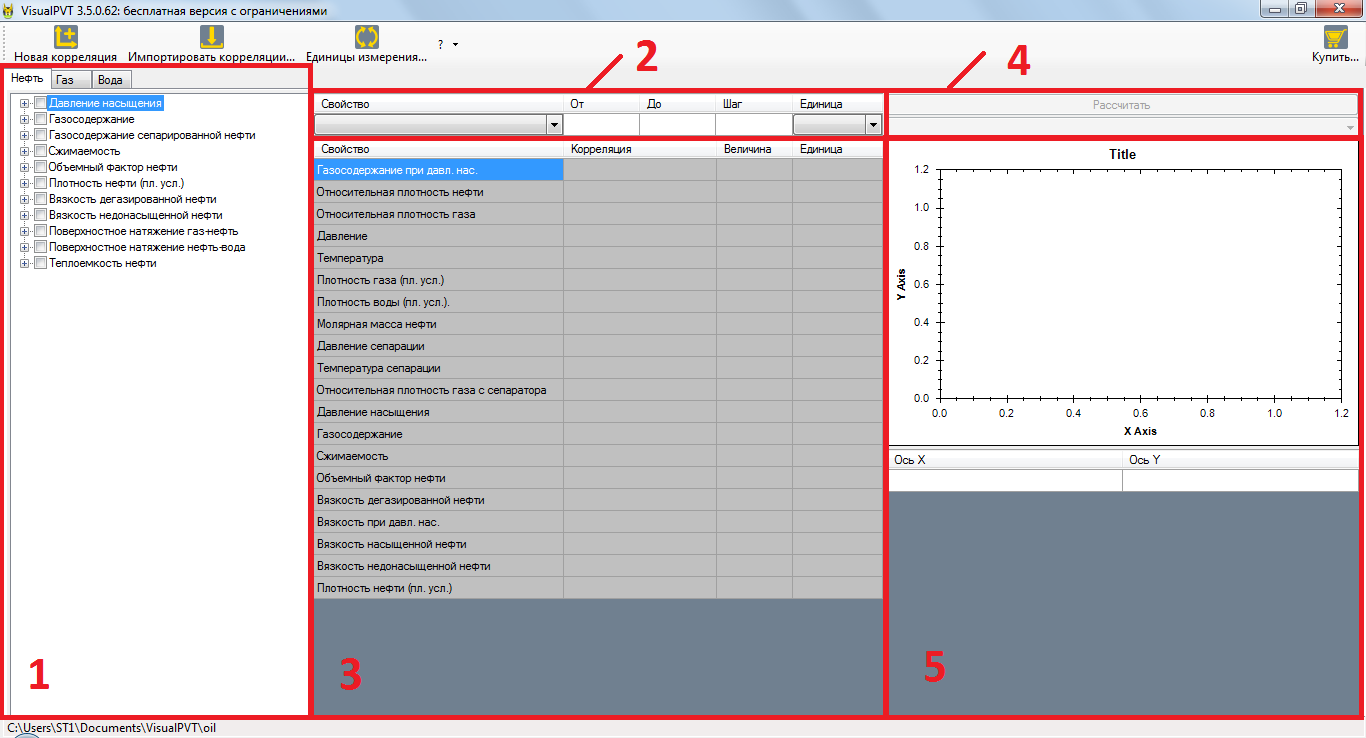1. "Oil", "Gas" and "Water" tabs are independent and have identical structure. Begin by selecting a tab of interest, then select a property of interest in the correlation tree. Up to this point, other Boxes of the main window remain inactive. The exception is the main menu buttons: their functionality will be described in the following sections of the documentation.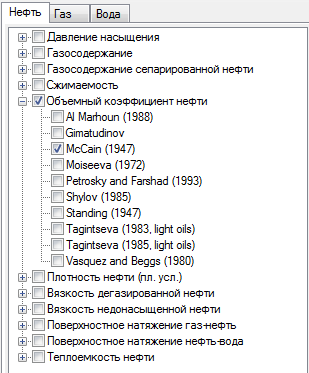You may select several properties at a time, but only the last one will be active. For example, if you check ?Gas-Oil Ratio? and ?Formation Volume Factor?, only the ?Formation Volume Factor? will be considered, as if ?Gas-Oil Ratio? were not checked at all (see the axes labels in the graph of Box 5):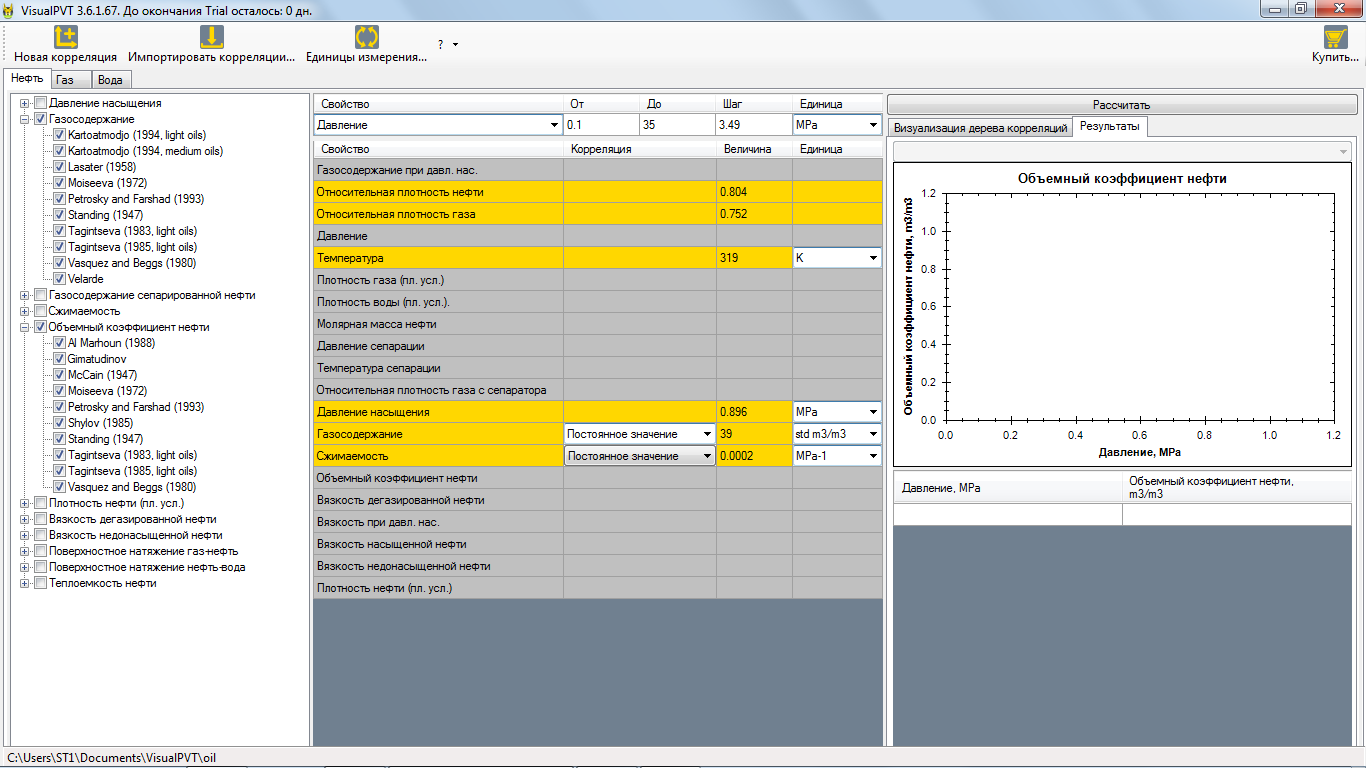You can select all correlations of a property by checking the corresponding PVT-property or select some of them by checking the correlations themselves.

2. When you have selected at least one correlation in the correlation tree, the table in Box 2 becomes active. Here you set a range of variation (tabulation) for one of the input parameters holding all other parameters fixed (see Box 3 below).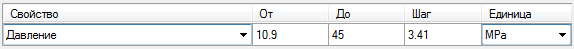If you selected only one correlation, the drop-down list contains the input parameters of this correlation. Otherwise, the drop-down list contains the intersection of the input parameters from all the selected correlations (in order for the tabulated parameter to be the input parameter for all of the calculated correlations). Note, the drop-down list may remain empty, then a warning message will be displayed.

The correlation validity limits (or, in the case of multiple correlations, the limits intersection) are used to set the ?From? and ?To? values. Correlation validity limits are published by the authors.

For a calculation to be performed, the "From? value must be less than or equal to the "To" value, and the ?Step? value must be more than zero: ?From? ? ?To?, "Step"> 0. If you need to calculate a value at one point, set "From" = "To."

The "Step" value is by default recalculated based on 10 points in the graph, and can always be adjusted.

3. Prior to calculation, in addition to selection of a parameter to be tabulated in Box 2, you should set values for other necessary parameters in the table of Box 3. When correlations are selected, a combination of all their input parameters is highlighted. When a parameter itself can be calculated from correlations, the second column of the corresponding raw will contain the drop-down list of these correlations. In the drop-down list, there is also an option to set a numerical value, selected by default.

By default, the values of necessary parameters are taken from the lower validity limits of correlations. When a correlation is selected, the value in column 3 is not required. It will be calculated along with the target property and updated after the calculation.

4. To start calculations, click on the ?Calculate? button. When clicked, a downstream drop-down list will be filled with the names of the correlations selected. Use the list to navigate through the Results Table below.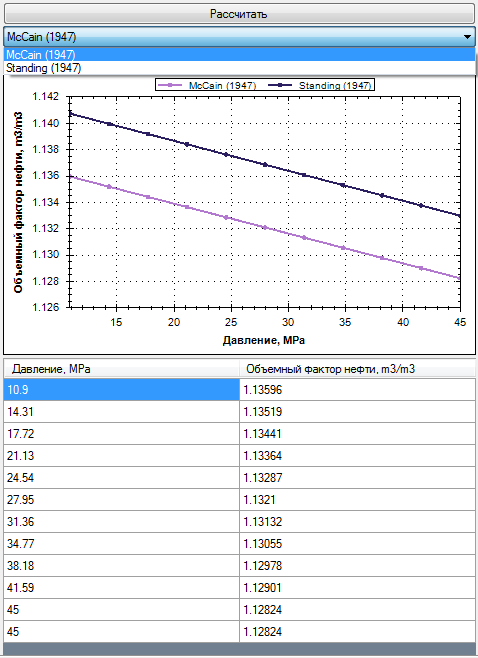In a given example, oil formation volume factor was plotted as a function of pressure at fixed values of gas-oil ratio (Rs) and compressibility (Co), set in the table of Box 3. In order for this function to be physically correct, Rs and Co should also be dependent on the tabulated pressure, which is illustrated in the Building the correlations tree section. That particular feature is one the most powerful and flexible capabilities of VisualPVT.

5. Using the right-click graph menu, you can save an image as a separate file as well as make use of other options.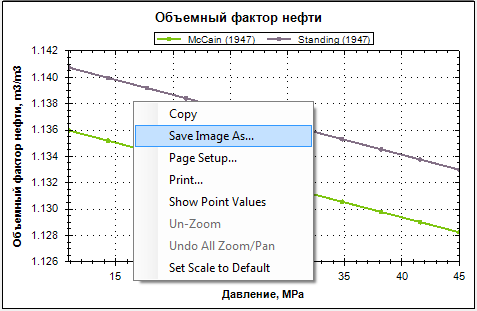In order to copy a table to clipboard, select any table row, press the key combination Ctrl+A and then Ctrl+C. After that, you can use Ctrl+V to paste the table content in any text editor or Word/Excel.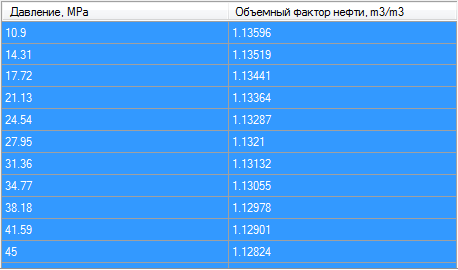Up

## Building the tree of correlations

Hereinafter, without loss of generality, a description for oil is given.

For example, oil bubble boint pressure and gas-oil ratio can be calculated based on a single correlation, because the input parameters of this correlation are user values of pressure, temperature, oil specific gravity, gas specific gravity, bubble point gas-oil ratio. Correlations for other properties may contain additional input parameters whose values are not known. They, in turn, can be calculated from correlations. It results in a tree of the correlation dependences that most closely corresponds to the PVT interdependences.

As an example, let us consider oil formation volume factor as a function of reservoir pressure. Check McCain correlation in the tree.The calculation of gas-oil ratio and compressibility from correlations is available now in the table of input parameters (Box 3).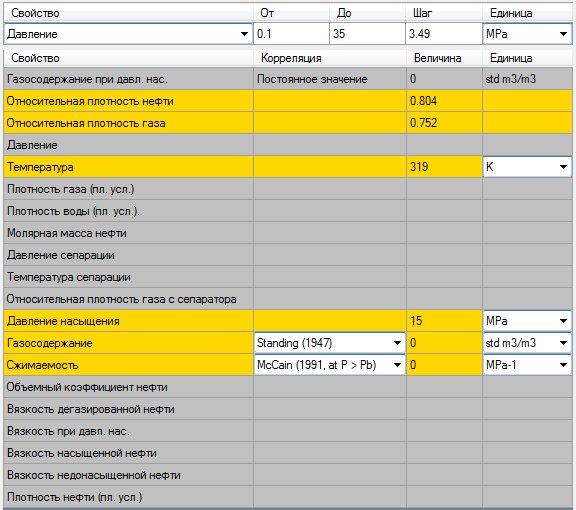If the ?Fixed value? option is selected, you should set a value in the ?Value? cell on the right. Otherwise, this field is not used. And, after calculation, this field will contain the last point value of the tabulated property (here, pressure point 35 Mpa).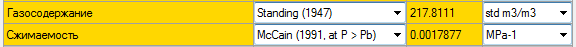Any change in the derived correlations are visualized on the graph and annotated. The annotations are self-explanatory.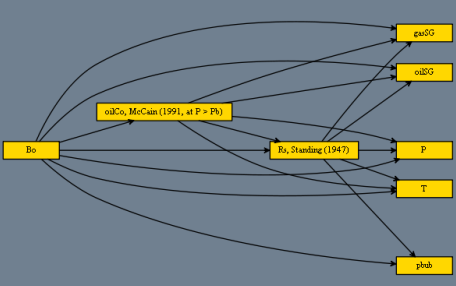Note, recursive dependences are avoided. That is, dependences, that are in turn dependent on the property selected in the correlations tree, are not added to the derived correlations list for input parameters. For example, Labedi (1994) correlation for compressibility involves the formation volume factor setting. Therefore, only Shylov (1985) correlation is included in the list of correlations available for calculation of the formation volume factor.

When derived correlations are selected, calculation results in regular function Bo(p) inflected at the bubble point pressure.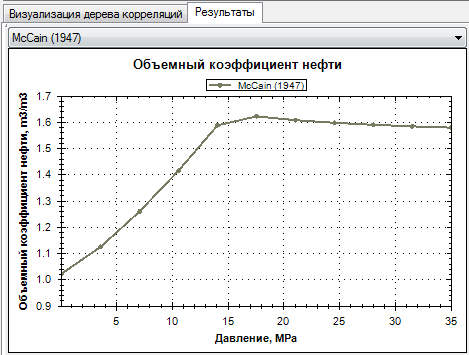Up